Courses

# Olympiad Test: Dice - 2

## 10 Questions MCQ Test Mathematics Olympiad Class 7 | Olympiad Test: Dice - 2

Description
This mock test of Olympiad Test: Dice - 2 for Class 7 helps you for every Class 7 entrance exam. This contains 10 Multiple Choice Questions for Class 7 Olympiad Test: Dice - 2 (mcq) to study with solutions a complete question bank. The solved questions answers in this Olympiad Test: Dice - 2 quiz give you a good mix of easy questions and tough questions. Class 7 students definitely take this Olympiad Test: Dice - 2 exercise for a better result in the exam. You can find other Olympiad Test: Dice - 2 extra questions, long questions & short questions for Class 7 on EduRev as well by searching above.
QUESTION: 1

### Two positions of a cube with its numbered surfaces are shown below. When the surface 4 touches the bottom, which surface will be on the top?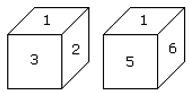Solution:

In these 2 positions one common face with number 1 is in the same position. Hence according to the rule number (3), 2 is opposite 6 and 3 is opposite to 5. Therefore opposite to 4 is 1.

QUESTION: 2

### Two positions of a dice are shown below. When number ‘1’ is on the top. What number will be at the bottom?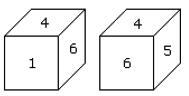Solution:

According to the rule (2) when ‘one’ is at the top, then 5 will be at the bottom.

QUESTION: 3

### Which number is on the face opposite to 6?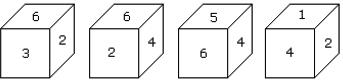Solution:

As the numbers 2, 3, 4 and 5 are adjacent to 6. Hence the number on the face opposite to 6 is 1.

QUESTION: 4

Six dice with upper faces erased are shown as: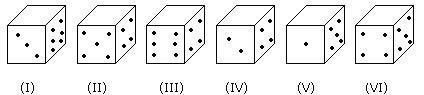The sum of the numbers of dots on the opposite face is 7.
Q. If even numbered dice have even number of dots on their top faces, then what would be the total number of dots on the top faces of their dice?

Solution:

Even numbered dice are: (II), (IV) and (VI)
No. of dots on the top face of (II) dice = 6
No. of dots on the top face of (IV) dice = 6
and No. of dots on the top face of (VI) dice = 6
Therefore required total = 6 + 6 + 6 = 18

QUESTION: 5

How many points will be on the face opposite to the face which contains 2 points?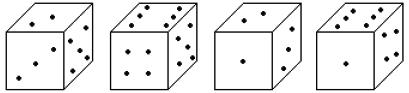Solution:

In first two positions of dice one common face containing 5 is same. Therefore according to Rule no. (3) the face opposite to the face which contains 2 point will contain 6 points.
A cube has six different symbols drawn over its six faces. The symbols are dot, circle, triangle, square, cross and arrow. Three different positions of the cube are shown in figures X, Y, and Z.

QUESTION: 6

Which symbol is opposite the arrow?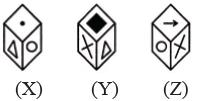Solution:

From figures X and Y, we conclude that dot, circle, square and cross lie adjacent to the triangle. Therefore, the arrow must lie opposite the triangle. From figures X and Z, we conclude that dot, triangle, arrow and cross lie adjacent to the circle. Therefore, the square must lie opposite the circle. Thus, the arrow lies opposite the triangle, the square lies opposite the circle and consequently, the cross lies opposite the dot.
As analysed above, the symbol opposite the arrow is triangle.

QUESTION: 7

Given below are three different positions of a dice. Find the number of dots on the face opposite the face bearing 3 dots.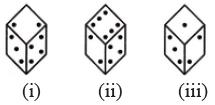Solution:

From figures (i), (ii) and (iii), we conclude that 3, 4, 6 and 1 dots appear adjacent to 2 dots. Therefore, 5 dots appear opposite 2 dots. Now, from figures (i) and (iii), we conclude that 2, 4 and 1 dots appear adjacent to 3 dots. Therefore, either 5 or 6 dots appear opposite 3 dots. Since, 5 dots appear opposite 2 dots, it follows that 6 dots appear opposite 3 dots.

QUESTION: 8

Which number is on the face opposite 4, if the four different positions of a dice are as shown in the figure given below.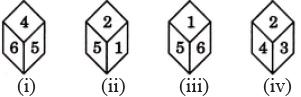Solution:

From figures (i) and (iv) we conclude that 6, 5, 2 and 3 lie adjacent to 4. It follows that 1 lies opposite 4.

QUESTION: 9

A cube has six different symbols drawn over its six faces. The symbols are dot, circle, triangle, square, cross and arrow. Three different positions of the cube are shown in figures X, Y, and Z.
Q. Which symbol occurs at the bottom of fig.(Y)?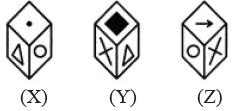Solution:

From figures X and Y, we conclude that dot, circle, square and cross lie adjacent to the triangle. Therefore, the arrow must lie opposite the triangle. From figures X and Z, we conclude that dot, triangle, arrow and cross lie adjacent to the circle. Therefore, the square must lie opposite the circle. Thus, the arrow lies opposite the triangle, the square lies opposite the circle and consequently, the cross lies opposite the dot.
Since the square lies at the top of figure (Y) and the circle lies opposite the square (as analysed above), so the circle occurs at the bottom of figure (Y).

QUESTION: 10

A dice is numbered from 1 to 6 in different ways.
Q. If 1 is adjacent to 2, 4 and 6, then which of the following statements is necessarily true?

Solution:

If 1 is adjacent to 2, 4 and 6, then either 3 or 5 lies opposite to 1. So, the numbers 3 and 5 cannot lie opposite to each other. Hence, 3 is adjacent to 5 (necessarily).